Monday 01st June 2020
 CBSE Guess > Papers > Question Papers > Class XII > 2005 > Physics > Delhi Set-III PHYSICS 2005 (Set III—Delhi)
Except for the following questions all the remaining questions have been asked in Set I and Set II.. Q. 1. Name an appropriate communication channel needed to send a signal of band-width 100 kHz over a distance of 8 km. 1 Q. 2. Give the direction in which the induced current flows in the coil mounted on an insulating stand when a bar magnet is quickly moved along the axis of the coil from one side to the other as shown in the figure. 1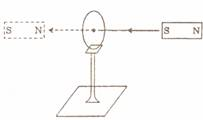Q. 3. Does the 'stopping potential' in photoelectric emission depend upon (i) the intensity of the incident radiation in a photocell? (ii) the frequency of the incident radiation? 1 Q. 4. An electron and a proton, moving parallel to each other in the same direction with equal momenta, enter into a uniform magnetic field which is at right angles to their velocities. Trace their trajectories in the magnetic field. 1 Q. 5. Define the term 'dielectric constant' of a medium. 1 Q. 7. Figure shows a bar magnet M falling under gravity through an air cored coil C. Plot a graph showing variation of induced e.m.f. (E) with time (t). What does the area enclosed by the E-t curve depict? 2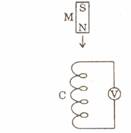Q. 13. In a metre bridge, the balance point is found to be at 39.5 cm from the end A, when the resistor Y is of 12.5 ohm. Determine the resistance of X. Why are the connections between resistors in a metre bridge made of thick copper strips? What happens if the galvanometer and cell are interchanged at the balance point of the bridge? Would the galvanometer show any current? 3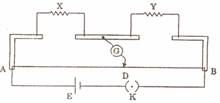Q. 16. Define the terms 'resistivity' and 'conductivity' and state their S. I . units. Draw a graph showing the variation of resistivity with temperature for a typical semiconductor. 3 Or State the principle of potentiometer. With the help of circuit diagram, describe a method to find the internal resistance of a primary cell. Q. 17. Three rays of light - red (R), green (C) and blue (B) - are incident on the face AB of a right-angled prism ABC. The refractive indices of the material of the prism for red, green and blue wavelengths are 1.39, 1.44 and 1.47 respectively. Trace the path of the rays through the prism. How will the situation change if these rays were incident normally on one of the faces of an equilateral prism?3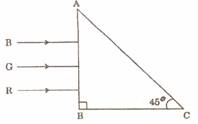Q. 21. Explain, with the help of a circuit diagram, how the thickness of depletion layer in a p-n junction diode changes when it is forward biased. In the follow- ing circuits which one of the two diodes is forward biased and which is reverse biased? 3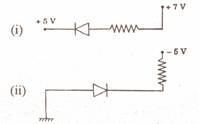Physics 2005 Question Papers Class XII Delhi Outside Delhi Compartment Delhi Compartment Outside Delhi Set I Set I Set I Set I Set II Set II Set II Set II Set III Set III Set III CBSE 2005 Question Papers Class XII